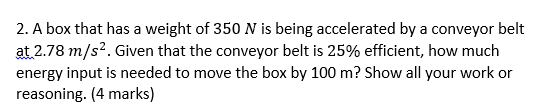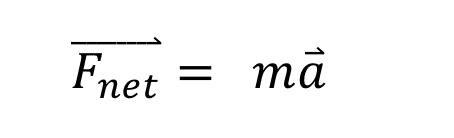# Efficiency and Energy to move a box on a conveyor belt

• UnknownQuestioner

#### UnknownQuestioner

New user has been reminded to show their work on schoolwork questions
Homework Statement
Please refer to the attached picture.
Relevant Equations
This is where I am stuck at, I can’t seem to figure out the formulas to use and the combination of using them.Last edited by a moderator:

What force acts on the box? I assume the conveyor belt is horizontal.

Gravity? Not sure

If the box is being accelerated by the belt, the belt's got to be exerting a force on it. You know the mass of the box (from the weight) and the acceleration, so can you get the force the belt exerts on the box?Yeah, but you can just use components here. Just say ##F=ma##. What is the force?

Would that be 350 x 2.78 to get 973 N as the force?

Would that be 350 x 2.78 to get 973 N as the force?

What's the mass of the box?

Oops, that would be 35.7 kg. Then 35.7 x 2.78 to get 99.2 N

Oops, that would be 35.7 kg. Then 35.7 x 2.78 to get 99.2 N
So how much work is done on the box in moving it the 100m?

So how much work is done on the box in moving it the 100m?

I used f=ma to get 99.2N then I used W=fd to get 9920J. This energy is the output, so output/0.25=input. To get a final answer of 3.97 x 10^4 J This is correct?

I used f=ma to get 99.2N then I used W=fd to get 9920J. This energy is the output, so output/0.25=input. To get a final answer of 3.97 x 10^4 J This is correct?
Looks good.